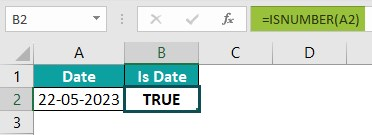# VBA Format DateArticle byJeevan A Y## Excel VBA Format Date

Date Format in VBA is a built-in function used to format dates in Excel. Excel is designed to work with data and data includes a lot of dates in it. We won’t get the data in ready format all the time. At times, we need to format the data to user ready and one such thing is formatting dates to fit with user readiness.

VBA allows us to automate the tasks and as part of automation, date formatting is also one task we should be aware of.

For example, look at the following data in Excel.

The above data shows the daily sales, however, when we look at the date format of yellow colored cells we have different formatting for dates. When we need to send out the sales summary to the top management of the company inconsistent date formatting won’t look good.

We can manually correct these inconsistent date formatting with small data, but it won’t be that easy with large data sets. In order to quickly format the dates we can use the VBA data formatting techniques.

###### Key Takeaways
• VBA Format Date is used to format date in excel.
• We can change the date formatting in VBA using the Number Formatting method and using the FORMAT function.
• Number Formatting and FORMAT functions are used to change the formatting of other numerical values also and are not limited only to date.
• We can format the date in any order such as dd-mm-yyyy or mm-dd-yyyy or yyyy-mm-dd.
• We can show the month name, and day name by using the custom formatting codes.

## Syntax Of VBA Date Format

We can use the FORMAT function in VBA to format the date. The following image shows the syntax of the VBA FORMAT function in Excel.

Expression: This is a compulsory argument. In this argument, we need to specify the date that we would like to format. The input could be a direct entry of date or it could be in the form of a variable or it could be in the form of excel cell reference as well.

[Format]: In this argument, we can specify the kind of date formatting that we need to apply to the given date in the first argument of the VAB Format function.

#### Pre-defined Date Formats

In this argument, we can give some of the following pre-defined date formats.

General Date

Short Date

Medium Date

Long Date

Apart from these pre-defined date formats, we can also give custom date formats like the following.

We can use various combination of formatting codes to get the desired date format in the given cell address. We will discuss all those things in this article.

### How to Change Date Format in VBA?

There are several ways we can change the date format in Excel using VBA. We will look at a few simple examples before we solve complex problems.

#### Example #1 – Change Date Format Using NumberFormat method in VBA

We can use the NumberFormat method in VBA to change the date format in Excel. For example, look at the following data we have in an Excel spreadsheet.

We have 3 dates, and all the three dates have inconsistent date format. To quickly fix the uniform date for these cells lets heard over to visual basic editor window by pressing the short cut key ALT + F11.

After pressing the short cut key ALT + F11 this will open the following visual basic editor window for us.

Start the subroutine by giving a name to the excel macro like the following one.

Step 1: First we need to apply the date formatting for cell A2. To reference the cell, enter the VBA RANGE object reference like the following.

Step 2: Enter the A2 cell reference in double quotes like the following.

Once the cell reference is given, enter the dot to see all the properties and methods associated with the RANGE object.

Step 3: Choose the NumberFormat property.

Step 4: After choosing the NumberFormat, enter an equal sign to set the number format of the given cell address.

Step 5: After the equal sign is entered, give the desired date format in double quotes. For example, if we want to show the date in date, month, and year format then enter the format code in double quotes like the following.

“dd-mm-yyyy”

Let’s execute the code by pressing the execution short cut key F5.

The date format changed to the given date format where we were able to see the month’s short name previously but now we can see the month in numbers. As we can see the difference between the old date format and the new date format in the above image.

#### Example 2

Continuing from the previous example, if we need to apply the same formatting for all the remaining cells, we cannot write VBA code for each line separately.

We need to use the loops to dynamically loop through all the cells and apply the formatting with a single line of code.

The following code will apply the consistent date format for all the cells.

Sub Date_Formatting_Ex2()
Dim k As Integer
Dim LR As Long
LR = Cells(Rows.Count, 1).End(xlUp).Row
For k = 2 To LR
Range(“A” & k).NumberFormat = “dd-mm-yyyy”
Next k
End Sub

Copy the above code to visual basic editor window.

Hit the green execute button in the visual basic editor window.

Once we execute the above code it will apply a consistent date format for all the cells.

Now all the cells have the same date format.

### Change Date Format by Using FORMAT Function

In the previous examples, we have seen changing the date format by using the Number Format property of the RANGE object.

Now, let us look at the ways of changing the date format by using the VBA FORMAT function.

#### Example #1 – Change the Date format By Using the Format Function

As we have discussed earlier we a have few built-in date formats like General Date, Short Date, Long Date, and Medium Date.

Let’s apply these built-in formatting using the FORMAT function.

Look at the following date value in Excel.

Step 1: Start the sub routine by naming the macro.

Step 2: Define a variable to store the value of cell A2.

Because we are going to store the date value to the variable we have given the data type as Date to the variable MyDate.

Step 3: Assign the value of cell A2 to the defined variable MyDate.

Step 4: Now we need to format this date to long date by using the format function. Since we need to store the value back to the same cell use the RANGE object and select the A2 cell reference with value property.

Step 5: Enter an equal sign and enter the FORMAT function like the following.

Step 6: The first argument requires the Expression i.e., the date that we are trying to change the format. A2 Cell value already assigned to the variable MyDate in Step 3. Enter the variable name for the expression argument of the FORMAT function.

Step 7: Next, give the format as Long Date in double quotes. The final code looks like the following.

Sub DateFormat()
Dim MyDate As Date
MyDate = Range(“A2”).Value
Range(“A2”).Value = Format(MyDate, “Long Date”)
End Sub

Run the code by pressing the shortcut key F5 and we will get the long date format in cell A2.

Like this we can play around with other built-in date formats as well.

#### Example #2 – Custom Date Formatting with the FORMAT Function in VBA

Most of the time we may end up doing the custom date formatting rather than the built-in date formatting.

Let us use different kinds of custom date formatting and see how it affects the rend result in Excel cell.

##### #1 – Show Only Day

Sub DateFormat_Day()
Dim MyDate As Date
MyDate = “25-11-2023”
Range(“A2”).Value = Format(MyDate, “dd”)
End Sub

Instead of providing the date through cell reference, we have directly assigned date to the variable directly and we have applied the formatting code “dd” to show only the day of the date.

Once we run the code it will store only the day of the date in cell A2 like the following.

Similarly the following codes will format the dates in various ways.

##### #2 – Show Month in Number

Code: Range(“A2”).Value = Format(MyDate, “mm”)

Result:

##### #3 – Show Month in Short Name

Code: Range(“A2”).Value = Format(MyDate, “mmm”)

Result:

##### #4 – Show Month in Full Name

Code: Range(“A2”).Value = Format(MyDate, “mmmm”)

Result:

##### #5 – Show Date in DD – MM – YYY Format

Code: Range(“A2”).Value = Format(MyDate, “dd-mm-yyyy”)

Result:

##### #6 – Show Date in MM – DD – YYYY Format

Code: Range(“A2”).Value = Format(MyDate, “mmm-dd-yyyy”)

Result:

##### #7 – Show Date in YYYY – MM – DD Format

Code: Range(“A2”).Value = Format(MyDate, “yyyy-mmm-dd”)

Result:

##### #8 – Show Year Only in 2 Digits

Code: Range(“A2”).Value = Format(MyDate, “dd-mm-yy “)

Result:

Like this, we can play around with various custom date formatting with the FORMAT function in VBA.

### Important Things to Note

• FORMAT function in VBA is not only used to change the date format but also used to change various numerical values formatting as well.
• In VBA FORMAT is a function and Number Formatting is a property.
• If the date is in cells reference and if it is stored as text whatever formatting, you apply will not be applicable for the given date.
• FORMAT is the function that is available only in VBA and you won’t find this under the worksheet function category.

1. Why is VBA Format Date is Not Working?

For example, look at the following date in Excel.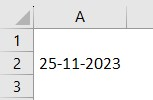If we apply the formatting for the above date it won’t work because the date is stored as text in cell A2.

To check if the value in the cell is actually a date or not we can use the ISNUMBER function in the worksheet.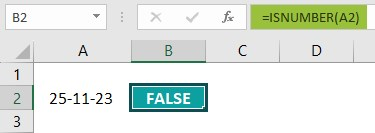In cell B2 we have applied the function ISNUMBER to check if the value in cell A2 is number or not. The formula has returned the result as FALSE i.e., the value in cell A2 is not a date rather it is a text. Hence, VBA format function cannot format this date.

2. How do I format a Date Column in Excel?

For example, look at the following date column in Excel.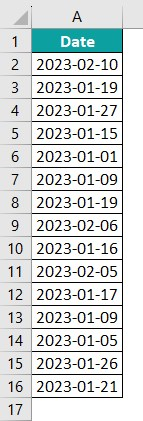The current date format is yyyy-mm-dd, to change the entire column date format to dd-mm-yyyy we can use the following code.

Sub DateFormat_EntireColumn()
Range(“A:A”).NumberFormat = “dd-mm-yyyy”
End Sub

In RANGE object we have given the entire column address as A:A.

This will change the date format to Date, Month, and Year like the following.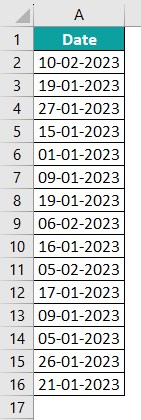3. How to Format Text Box Date as Date?

For example, look at the following date cell in Excel.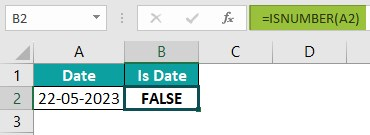The A2 cell value is not date because ISNUMBER function in cell B2 returned FALSE result.

The value is date looking text value, hence we can use the following code to convert to date.

Sub DateFormat_Text()
Dim Original_Value As Variant
Original_Value = Range(“A2”).Value
Dim Target_Value As Long
Target_Value = CDate(Original_Value)
Range(“A2”).Value = CDate(Format(Target_Value, “dd-mm-yyyy”))
End Sub

The above code has returned TRUE value as shown.